# E-PolyLearning

 1. Overall distillation column efficiency for most of the refinery columns can be given by (for μ = 0.07 - 1.4 cp and relative volatility < 4) (where η = overall column efficiency, % μ = molal average viscosity of feed at average column temperature and pressure, cp Δp = pressure drop per tray, psi)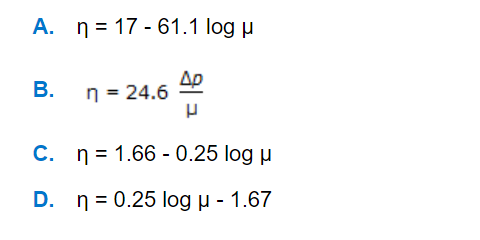a. A b. B c. C d. D

 2. For laminar flow (Nʀₑ < 2100) in steel pipe, the optimum inside pipe diameter is given by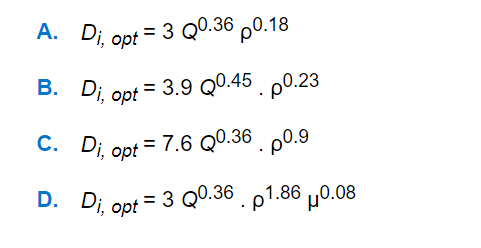a. A b. B c. C d. D
 3. For annular flow of a fluid, the ratio of the equivalent diameter for pressure drop calculation to the equivalent diameter for heat transfer calculation is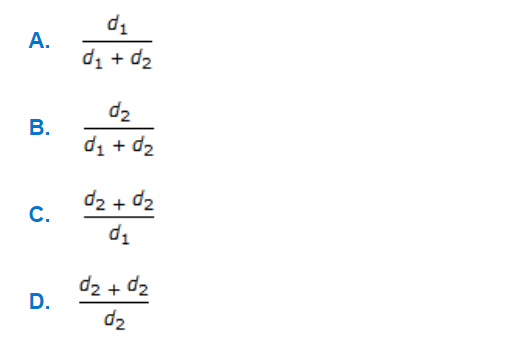a. A b. B c. C d. D
 4. Pressure drop due to pipe fittings can be estimated by the following equation, where, Lₑ = equivalent length of straight pipeline which will incur the same frictional loss as the fitting and D = diameter of the fitting. The value of Lₑ/D (dimensionless) for 45° elbow and 180° close return bends would be respectively around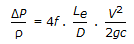a. 5 and 10 b. 45 and 75 c. 180 and 300 d. 300 and 500
 5. In a rotary drier, the average retention time of solid is (where Z = length of the drier, metres ρ = apparent solid density, kg/m³ L = flow rate of dry solids, kg/see. m² drier cross-section H = hold up of solid.)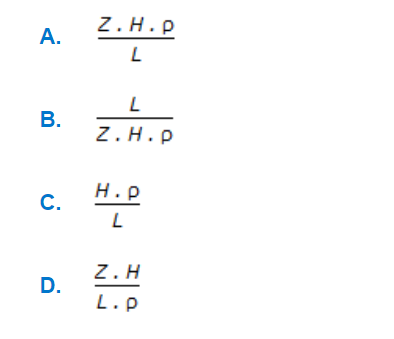a. A b. B c. C d. D